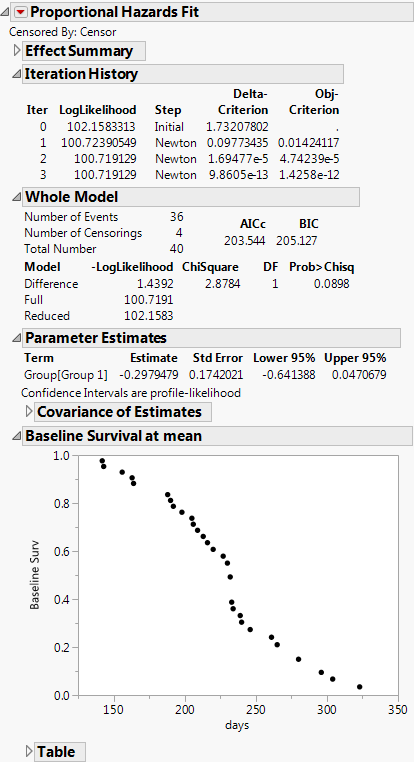Reliability and Survival Methods > Fit Proportional Hazards > The Fit Proportional Hazards Report
Publication date: 08/13/2020

# The Fit Proportional Hazards Report

Finding parameter estimates for a proportional hazards model is an iterative procedure. When the fitting is complete, the report in Figure 15.5 appears.

Figure 15.5 The Proportional Hazards Fit ReportIteration History

Lists iteration results occurring during the model calculations.

Whole Model

Shows the negative of the log-likelihood function (–LogLikelihood) for the model with and without the covariates. Twice the positive difference between them gives a chi-square test of the hypothesis that there is no difference in survival time among the effects. The degrees of freedom (DF) are equal to the change in the number of parameters between the full and reduced models.

Parameter Estimates

Shows the parameter estimates for the covariates, their standard errors, and 95% upper and lower confidence limits. A confidence interval for a continuous column that does not include zero indicates that the effect is significant. A confidence interval for a level in a categorical column that does not include zero indicates that the difference between the level and the average of all levels is significant.

Effect Likelihood Ratio Tests

Shows the likelihood ratio chi-square test of the null hypothesis that the parameter estimates for the effects of the covariates is zero.

Baseline Survival at mean

Plots the baseline function estimates at each event time in the data. The values in the Table report are plotted here.